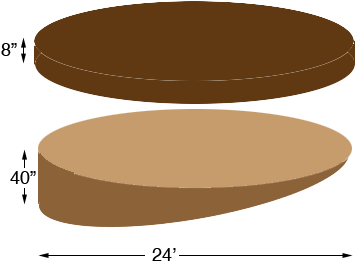SEARCH HOMEMath Central Quandaries & QueriesQuestion from Tim, a parent: Dear Sirs I have to fill a hole with clay and then 8 inches of top soil. The area is a 24 foot circular hole left by a now removed swimming pool. The area in question pitches from the house from 48" to ground level within that 24 feet. I was wondering how many yards o the top soil has to be 5-8 inches deep thank you f fill(clay) I would need and how many Yards of top soil to cover that holeTim,

I would use an approximation to make the calculation easier. My approximation is that the topsoil is a cylinder od diameter 24 feet and thickness 8 inches and the fill is half a cylinder of radius 24 feet and depth 40 inches.The volume of a cylinder is π r2 h where r is the radius and h is the height. You want the volume in cubic yards so change the dimensions to yards before making the calculation.

Topsoil: r = 12/3 = 4 yards; h = 8/36 yards: Volume = π × 42 × 8/36 = 11 cubic yards

Fill: r = 12/3 = 4 yards: h = 40/36 yards; Volume = 1/2 × π × 42 × 40/36 = 28 cubic yards.

HarleyMath Central is supported by the University of Regina and The Pacific Institute for the Mathematical Sciences.